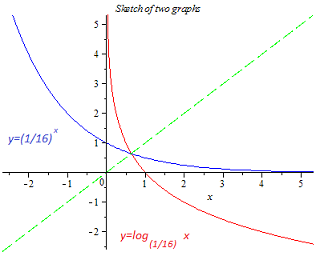# Logarithm-exponential Equation Conundrum

Calculus Level 5

Ahmad was trying to find the number of solutions to

$\log_{(1/16)}x=\left(\frac{1}{16}\right)^x.$

He noticed that these functions are inverses of each other. He drew a sketch of the function $y = \log_{(1/16)}x$ in red, and then flipped it about the line $y=x$ (shown in dotted green), to obtain a sketch of the function $y=\left(\frac{1}{16}\right)^x$ in blue.The picture suggests that the equation has one real solution. But algebra suggests that there are two: $x=\frac{1}{4}$ and $x=\frac{1}{2}$. How many real solutions does this equation actually have?

×

Problem Loading...

Note Loading...

Set Loading...